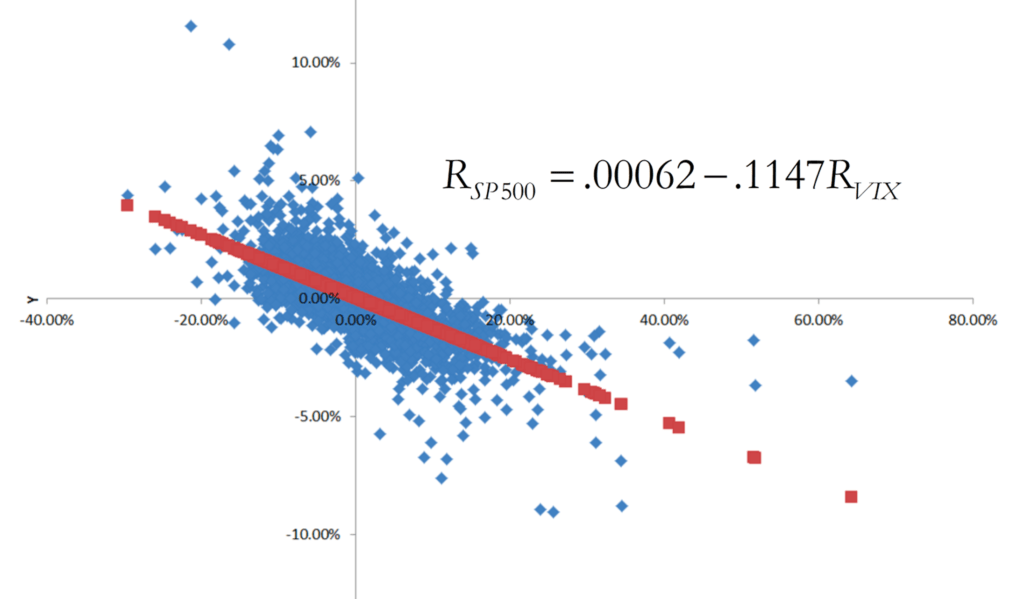## On the relationship between the S&P 500 and the CBOE Volatility Index (VIX)

Besides going over the course syllabus during the first day of class on Tuesday, January 17, we will also discuss a particularly important “real world” example of financial risk. Specifically, we will study the relationship between realized daily stock market returns (as measured by daily percentage changes in the SP500 stock market index) and changes in forward-looking investor expectations of stock market volatility (as indicated by daily percentage changes in the CBOE Volatility Index (VIX)):As indicated by this graph (which also appears in the lecture note for the first day of class), daily percentage changes on closing prices for the SP500 (the y-axis variable) and for the VIX (the x-axis variable) are strongly negatively correlated with each other. The blue dots are based on 8,315 contemporaneous observations of daily returns for both variables, spanning the 33-year period of time starting on January 2, 1990 and ending on December 30, 2022. When we fit a regression line through this scatter diagram, we obtain the following equation:${R_{SP500}} = .00062 - .1147{R_{VIX}}$,

where${R_{SP500}}$ corresponds to the daily return on the SP500 index and${R_{VIX}}$ corresponds to the daily return on the VIX index. The slope of this line (-0.1147) indicates that on average, daily closing SP500 returns are inversely related to daily closing VIX returns.  Furthermore, nearly half of the variation in the stock market return during this time period (specifically, 48.87%) can be statistically “explained” by changes in volatility, and the correlation between${R_{SP500}}$ and${R_{VIX}}$ came out to -0.70. While a correlation of -0.70 does not imply that daily closing values for${R_{SP500}}$ and${R_{VIX}}$ always move in opposite directions, it does suggest that this will be the case more often than not. Indeed, closing daily values recorded for${R_{SP500}}$ and${R_{VIX}}$ during this period moved inversely 78.59% of the time.